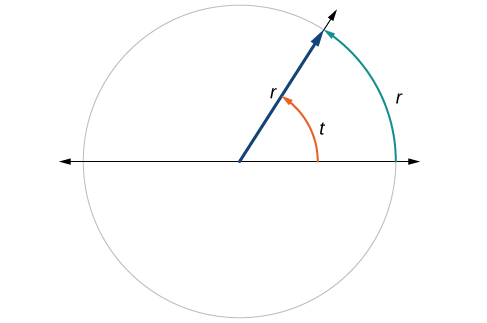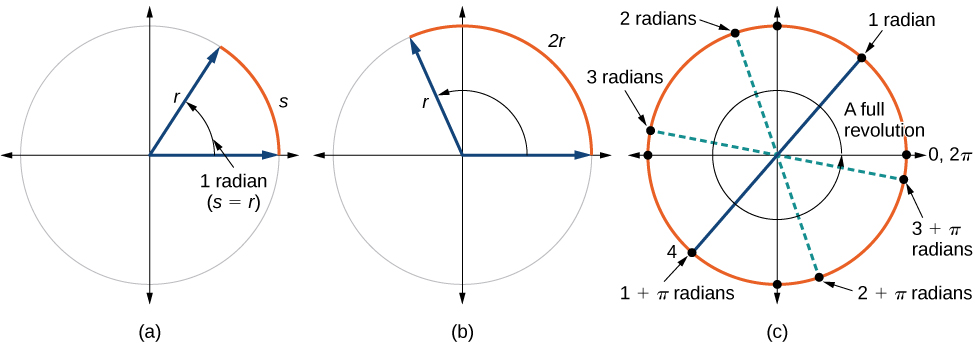# 7.1 Angles  (Page 3/29)

 Page 3 / 29

This brings us to our new angle measure. One radian    is the measure of a central angle of a circle that intercepts an arc equal in length to the radius of that circle. A central angle is an angle formed at the center of a circle by two radii. Because the total circumference equals $\text{\hspace{0.17em}}2\pi \text{\hspace{0.17em}}$ times the radius, a full circular rotation is $\text{\hspace{0.17em}}2\pi \text{\hspace{0.17em}}$ radians.

See [link] . Note that when an angle is described without a specific unit, it refers to radian measure. For example, an angle measure of 3 indicates 3 radians. In fact, radian measure is dimensionless, since it is the quotient of a length (circumference) divided by a length (radius) and the length units cancel.The angle   t   sweeps out a measure of one radian. Note that the length of the intercepted arc is the same as the length of the radius of the circle.

## Relating arc lengths to radius

An arc length     $\text{\hspace{0.17em}}s\text{\hspace{0.17em}}$ is the length of the curve along the arc. Just as the full circumference of a circle always has a constant ratio to the radius, the arc length produced by any given angle also has a constant relation to the radius, regardless of the length of the radius.

This ratio, called the radian measure, is the same regardless of the radius of the circle—it depends only on the angle. This property allows us to define a measure of any angle as the ratio of the arc length $\text{\hspace{0.17em}}s\text{\hspace{0.17em}}$ to the radius r . See [link] .

$\begin{array}{ccc}s& =& r\theta \\ \theta & =& \frac{s}{r}\end{array}$

If $\text{\hspace{0.17em}}s=r,$ then(a) In an angle of 1 radian, the arc length   s   equals the radius   r .   (b) An angle of 2 radians has an arc length   s = 2 r .   (c) A full revolution is   2 π , or about 6.28 radians.

To elaborate on this idea, consider two circles, one with radius 2 and the other with radius 3. Recall the circumference of a circle is $\text{\hspace{0.17em}}C=2\pi r,$ where $\text{\hspace{0.17em}}r\text{\hspace{0.17em}}$ is the radius. The smaller circle then has circumference $\text{\hspace{0.17em}}2\pi \left(2\right)=4\pi \text{\hspace{0.17em}}$ and the larger has circumference $\text{\hspace{0.17em}}2\pi \left(3\right)=6\pi .\text{\hspace{0.17em}}$ Now we draw a $\text{\hspace{0.17em}}45°\text{\hspace{0.17em}}$ angle on the two circles, as in [link] .

Notice what happens if we find the ratio of the arc length divided by the radius of the circle.

Since both ratios are $\text{\hspace{0.17em}}\frac{1}{4}\pi ,$ the angle measures of both circles are the same, even though the arc length and radius differ.

One radian    is the measure of the central angle of a circle such that the length of the arc between the initial side and the terminal side is equal to the radius of the circle. A full revolution $\text{\hspace{0.17em}}\left(360°\right)\text{\hspace{0.17em}}$ equals $\text{\hspace{0.17em}}2\pi \text{\hspace{0.17em}}$ radians. A half revolution $\text{\hspace{0.17em}}\left(180°\right)\text{\hspace{0.17em}}$ is equivalent to $\text{\hspace{0.17em}}\pi \text{\hspace{0.17em}}$ radians.

The radian measure    of an angle is the ratio of the length of the arc subtended by the angle to the radius of the circle. In other words, if $\text{\hspace{0.17em}}s\text{\hspace{0.17em}}$ is the length of an arc of a circle, and $\text{\hspace{0.17em}}r\text{\hspace{0.17em}}$ is the radius of the circle, then the central angle containing that arc measures $\text{\hspace{0.17em}}\frac{s}{r}\text{\hspace{0.17em}}$ radians. In a circle of radius 1, the radian measure corresponds to the length of the arc.

A measure of 1 radian looks to be about $\text{\hspace{0.17em}}60°.\text{\hspace{0.17em}}$ Is that correct?

Yes. It is approximately $\text{\hspace{0.17em}}57.3°.\text{\hspace{0.17em}}$ Because $\text{\hspace{0.17em}}2\pi \text{\hspace{0.17em}}$ radians equals $360°,1$ radian equals $\text{\hspace{0.17em}}\frac{360°}{2\pi }\approx 57.3°.$

(x2-2x+8)-4(x2-3x+5)
sorry
Miranda
x²-2x+9-4x²+12x-20 -3x²+10x+11
Miranda
x²-2x+9-4x²+12x-20 -3x²+10x+11
Miranda
(X2-2X+8)-4(X2-3X+5)=0 ?
master
The anwser is imaginary number if you want to know The anwser of the expression you must arrange The expression and use quadratic formula To find the answer
master
The anwser is imaginary number if you want to know The anwser of the expression you must arrange The expression and use quadratic formula To find the answer
master
Y
master
master
Soo sorry (5±Root11* i)/3
master
Mukhtar
explain and give four example of hyperbolic function
What is the correct rational algebraic expression of the given "a fraction whose denominator is 10 more than the numerator y?
y/y+10
Mr
Find nth derivative of eax sin (bx + c).
Find area common to the parabola y2 = 4ax and x2 = 4ay.
Anurag
A rectangular garden is 25ft wide. if its area is 1125ft, what is the length of the garden
to find the length I divide the area by the wide wich means 1125ft/25ft=45
Miranda
thanks
Jhovie
What do you call a relation where each element in the domain is related to only one value in the range by some rules?
A banana.
Yaona
given 4cot thither +3=0and 0°<thither <180° use a sketch to determine the value of the following a)cos thither
what are you up to?
nothing up todat yet
Miranda
hi
jai
hello
jai
Miranda Drice
jai
aap konsi country se ho
jai
which language is that
Miranda
I am living in india
jai
good
Miranda
what is the formula for calculating algebraic
I think the formula for calculating algebraic is the statement of the equality of two expression stimulate by a set of addition, multiplication, soustraction, division, raising to a power and extraction of Root. U believe by having those in the equation you will be in measure to calculate it
Miranda
state and prove Cayley hamilton therom
hello
Propessor
hi
Miranda
the Cayley hamilton Theorem state if A is a square matrix and if f(x) is its characterics polynomial then f(x)=0 in another ways evey square matrix is a root of its chatacteristics polynomial.
Miranda
hi
jai
hi Miranda
jai
thanks
Propessor
welcome
jai
What is algebra
algebra is a branch of the mathematics to calculate expressions follow.
Miranda
Miranda Drice would you mind teaching me mathematics? I think you are really good at math. I'm not good at it. In fact I hate it. 😅😅😅
Jeffrey
lolll who told you I'm good at it
Miranda
something seems to wispher me to my ear that u are good at it. lol
Jeffrey
lolllll if you say so
Miranda
but seriously, Im really bad at math. And I hate it. But you see, I downloaded this app two months ago hoping to master it.
Jeffrey
which grade are you in though
Miranda
oh woww I understand
Miranda
Jeffrey
Jeffrey
Miranda
how come you finished in college and you don't like math though
Miranda
gotta practice, holmie
Steve
if you never use it you won't be able to appreciate it
Steve
I don't know why. But Im trying to like it.
Jeffrey
yes steve. you're right
Jeffrey
so you better
Miranda
what is the solution of the given equation?
which equation
Miranda
I dont know. lol
Jeffrey
Miranda
Jeffrey
answer and questions in exercise 11.2 sums
how do u calculate inequality of irrational number?
Alaba
give me an example
Chris
and I will walk you through it
Chris
cos (-z)= cos z .
cos(- z)=cos z
Mustafa
what is a algebra
(x+x)3=?
6x
Obed
what is the identity of 1-cos²5x equal to?
__john __05
Kishu
Hi
Abdel
hi
Ye
hi
Nokwanda
C'est comment
Abdel
Hi
Amanda
hello
SORIE
Hiiii
Chinni
hello
Ranjay
hi
ANSHU
hiiii
Chinni
h r u friends
Chinni
yes
Hassan
so is their any Genius in mathematics here let chat guys and get to know each other's
SORIE
I speak French
Abdel
okay no problem since we gather here and get to know each other
SORIE
hi im stupid at math and just wanna join here
Yaona
lol nahhh none of us here are stupid it's just that we have Fast, Medium, and slow learner bro but we all going to work things out together
SORIE
it's 12ByByBy OpenStaxBy Robert MorrisBy OpenStaxBy OpenStaxBy Yasser IbrahimBy OpenStaxBy Jessica CollettBy Rohini AjayBy Brooke DelaneyBy Christine Zeelie